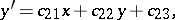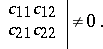# Affine transformation

(diff) ← Older revision | Latest revision (diff) | Newer revision → (diff)

of a Euclidean space

A one-to-one point mapping of the plane or space into itself, where to three points lying on a straight line correspond three points also lying on a straight line. Thus, by an affine transformation straight lines are transformed into straight lines. An affine transformation of the plane transforms intersecting lines into intersecting lines, and parallel lines into parallel lines. An affine transformation of space results in an affine mapping of each plane onto some plane; intersecting planes are mapped into intersecting planes, while parallel planes are mapped into parallel planes. Moreover, the mutual location of two straight lines is preserved: intersecting straight lines are mapped into intersecting straight lines, parallel straight lines are mapped into parallel straight lines, while skew lines are mapped into skew lines.

Under an affine transformation the ratio between directed segments lying on the same line or on parallel straight lines is equal to the ratio of their images. The ratio between the surface areas of two measurable figures (on the Euclidean plane) and the ratio between the volumes of two measurable bodies (in the Euclidean space) are preserved as well. Under an affine transformation a set of vectors in the plane (in space) is one-to-one mapped on a set of vectors in the plane (in space), and this mapping is linear. An affine transformation is defined in an affine coordinate system by a non-degenerate (non-homogeneous) linear transformation; thus, in the case of a plane, an affine transformation is analytically expressed by the formulaswith the supplementary conditionAffine transformations in space are defined in a similar manner.

Under an affine transformation an algebraic curve is converted into an algebraic curve, the order of the curves being preserved. In particular, a curve of the second order is converted into a curve of the second order, moreover, ellipses are converted into ellipses, hyperbolas into hyperbolas, parabolas into parabolas, etc.

Examples of affine transformations: isometric transformations, similarity transformations, and uniform contractions of a plane to a straight line. Each affine transformation in a plane is a product of an isometric transformation and two uniform contractions to two mutually-perpendicular straight lines. Each affine transformation in a space is a product of an isometric transformation and three uniform contractions to three pairwise-perpendicular planes.

The affine transformations form a group; the similarity transformations constitute a subgroup of this group; the set of isometric transformations is a subgroup of the group of similarity transformations.

Affine transformations are the most general one-to-one mappings of a plane (a space) into itself under which straight lines are preserved.